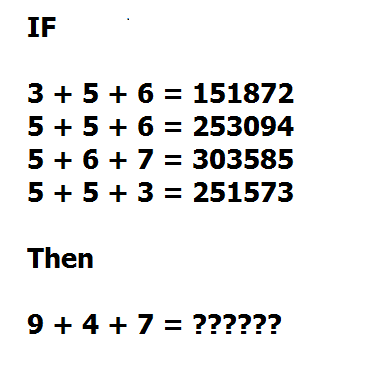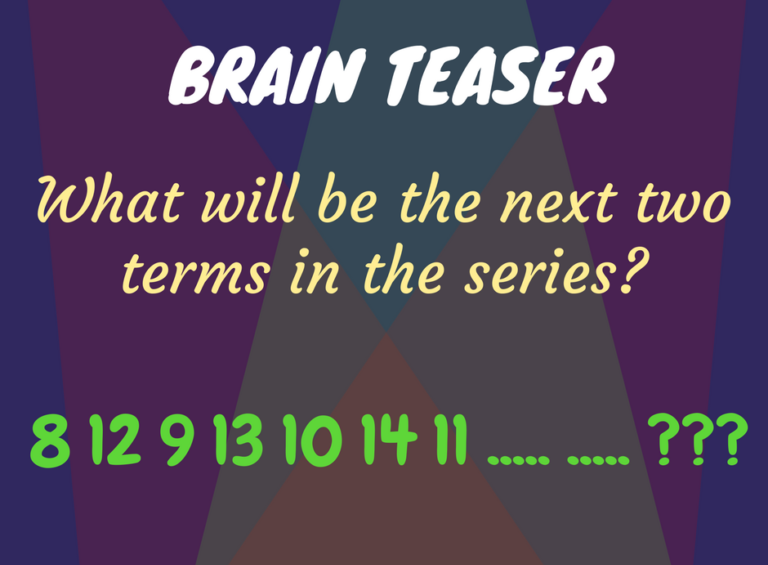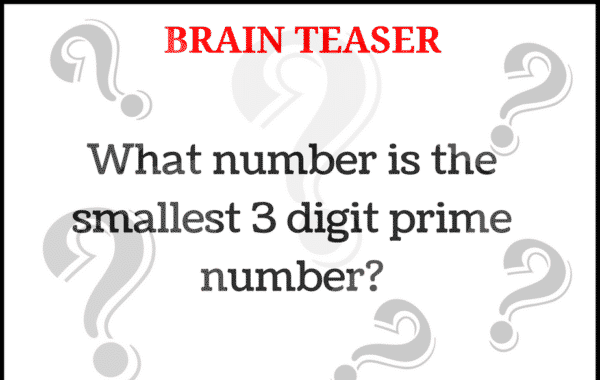The Brain Booster

The Brain Game – Booster

Check your mental ability right now… Here are some tricky questions with images check your brain power. All the answer are given below at the end of the post.

Brain Game – 1Brain Game – 2Brain Game – 3Brain Game – 4Brain Game – 5Brain Game – 6Brain Game – 7Brain Game – 8Brain Game – 9Here is the Answer with Explanation

1. 87 – The trick is to reverse the Image and See. (Rotate your Phone)

2. 211 – In this addition series, 1 is added to the first number 2 is added to the second number 3 is added to the third number 4 is added to the fourth number and go on.

3. 12- All the months have 28 days.

4. 366329 – First two digit =  first number * second number

5. 10 – This is a simple alternating addition and subtraction series. In the first pattern, 3 is added; in the second, 2 is subtracted.

6. 15 and 12 – This alternating addition series begins with 8; then 1 is added to give 9; then 1 is added to give 10; and so on.

7. The numbers, which are in color attract the eye & the reader may automatically find themselves checking those for a mistake.  In fact, the error is hidden in the text that is “Can you find the the Mistake”

8. 101 –

101 < 11 and 101 are not divisible by any of the prime numbers 2, 3, 5, 7, 11. 101 is a prime number. Hence 101 is the smallest 3-digit prime number.

9. 14 – This is a simple alternating addition and subtraction series. The first series begins with 8 and adds 3; the second begins with 43 and subtracts 2.